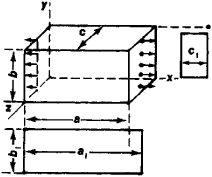# Poisson's Ratio

(redirected from Poison's ratio)
Also found in: Dictionary.

## Poisson’s Ratio

one of the physical quantities characterizing an elastic solid. It is equal to the ratio of the absolute value of the lateral strain to the absolute value of the longitudinal strain and was introduced by S. D. Poisson.

Suppose a rectangular parallelepiped is stretched in the direction of the x-axis (Figure 1). The parallelepiped undergoes an elongation of ∈x = (a1a)/a > 0 along this axis and a contraction of ∈y = (b1b)/b < 0 and ∈z = (c1c)/c < 0 along the perpendicular y-axis and z-axis, respectively—in other words, its lateral cross section shrinks. Poisson’s ratio is equal to vyx = ǀ∈y ǀ/ǀ∈x ǀor vzx = ǀ∈zǀ/ǀcǀ.Figure 1

In the case of an isotropic solid, Poisson’s ratio remains invariant if tension is replaced by compression or if the strain axes are changed—that is, vxy = νyx = vzx = v. Poisson’s ratio for anisotropic bodies is a function of the direction of the axes—that is, νxyvyxvzx. Poisson’s ratio, together with one of the moduli of elasticity, determines all the elastic properties of an isotropic solid. For most metals, Poisson’s ratio is close to 0.3.

## poisson’s ratio

In a material under tension or compression, the absolute value of the ratio of transverse strain to the corresponding longitudinal strain.
Site: Follow: Share:
Open / Close